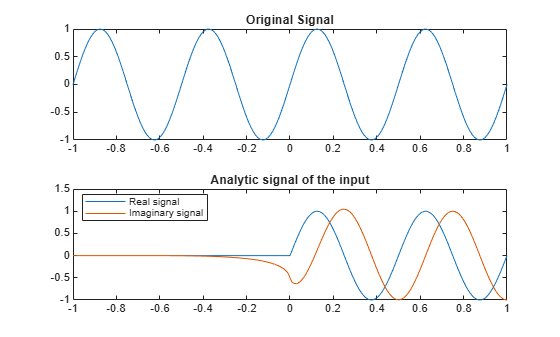Documentation

# dsp.AnalyticSignal

Analytic signals of discrete-time inputs

## Description

The `dsp.AnalyticSignal` System object™ computes analytic signals of discrete-time inputs. The real part of the analytic signal in each channel is a replica of the real input in that channel, and the imaginary part is the Hilbert transform of the input. In the frequency domain, the analytic signal doubles the positive frequency content of the original signal while zeroing-out negative frequencies and retaining the DC component. The object computes the Hilbert transform using an equiripple FIR filter.

To compute the analytic signal of a discrete-time input:

1. Create the `dsp.AnalyticSignal` object and set its properties.

2. Call the object with arguments, as if it were a function.

## Creation

### Syntax

``anaSig = dsp.AnalyticSignal``
``anaSig = dsp.AnalyticSignal(order)``
``anaSig = dsp.AnalyticSignal(Name,Value)``

### Description

````anaSig = dsp.AnalyticSignal` returns an analytic signal object, `anaSig`, that computes the complex analytic signal corresponding to each channel of a real M-by-N input matrix.```

example

````anaSig = dsp.AnalyticSignal(order)` returns an analytic signal object, `anaSig`, with the FilterOrder property set to `order`.```
````anaSig = dsp.AnalyticSignal(Name,Value)` returns an analytic signal object, `anaSig`, with each specified property set to the specified value.```

## Properties

expand all

Unless otherwise indicated, properties are nontunable, which means you cannot change their values after calling the object. Objects lock when you call them, and the `release` function unlocks them.

If a property is tunable, you can change its value at any time.

Order of the equiripple FIR filter used in computing the Hilbert transform, specified as an even integer scalar greater than 3.

Data Types: `single` | `double` | `int8` | `int16` | `int32` | `int64` | `uint8` | `uint16` | `uint32` | `uint64`

## Usage

### Syntax

``y = anaSig(x)``

### Description

example

````y = anaSig(x)` computes the analytic signal, `y`, of the M-by-N input matrix `x`, according to the equation $Y=X+jH\left\{X\right\}$ where j is the imaginary unit and $H\left\{X\right\}$ denotes the Hilbert transform.Each of the N columns in `x` contains M sequential time samples from an independent channel. The method computes the analytic signal for each channel.```

### Input Arguments

expand all

Data input, specified as a vector or a matrix.

Data Types: `single` | `double`
Complex Number Support: Yes

### Output Arguments

expand all

Analytic signal output, returned as a vector or a matrix.

Data Types: `single` | `double`
Complex Number Support: Yes

## Object Functions

To use an object function, specify the System object as the first input argument. For example, to release system resources of a System object named `obj`, use this syntax:

`release(obj)`

expand all

 `step` Run System object algorithm `release` Release resources and allow changes to System object property values and input characteristics `reset` Reset internal states of System object

## Examples

expand all

Note: This example runs only in R2016b or later. If you are using an earlier release, replace each call to the function with the equivalent `step` syntax. For example, myObject(x) becomes step(myObject,x).

Compute the analytic signal of a sinusoidal input.

```t = (-1:0.01:1)'; x = sin(4*pi*t); anaSig = dsp.AnalyticSignal(200); y = anaSig(x);```

View the analytic signal.

```subplot(2,1,1); plot(t, x) title('Original Signal'); subplot(2,1,2), plot(t, [real(y) imag(y)]); title('Analytic signal of the input') legend('Real signal','Imaginary signal',... 'Location','best');```## Algorithms

This object implements the algorithm, inputs, and outputs described on the Analytic Signal block reference page. The object properties correspond to the block parameters.